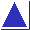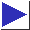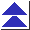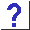# comp.lang.c FAQ list ·Question 3.3b

Q: Here's a slick expression:

`a ^= b ^= a ^= b`
It swaps a and b without using a temporary.

A: Not portably, it doesn't. It attempts to modify the variable a twice between sequence points, so its behavior is undefined.

For example, it has been reported that when given the code

```	int a = 123, b = 7654;
a ^= b ^= a ^= b;
```
the SCO Optimizing C compiler (icc) sets b to 123 and a to 0.

See also questions 3.1, 3.8, 10.3, and 20.15c.